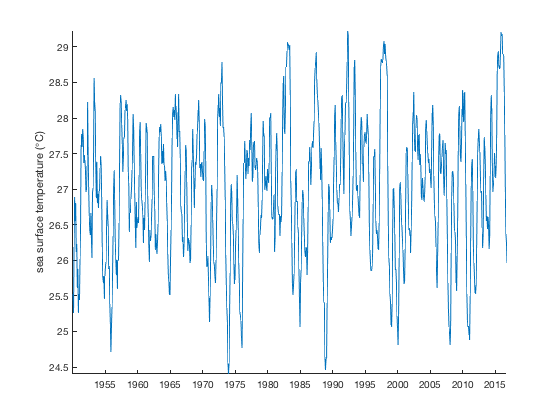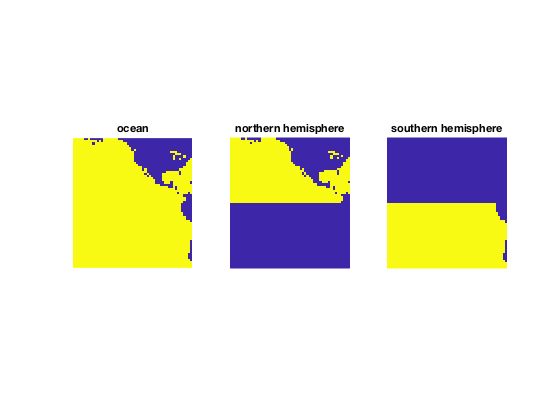# local documentation

The local function returns a 1D array of values calculated from a region of interest in a 3D matrix. For example, if you have a big global 3D sea surface temperature dataset, this function makes it easy to obtain a time series of the average sst within a region of interest.

Back to Climate Data Tools Contents

## Syntax

y = local(A)
y = local(...,FunctionInputs,...)
y = local(...,'omitnan')

## Description

y = local(A) produces a time series or profile from the mean values of each 2D slice along dimension 3 of the 3D matrix A.

y = local(A,mask) gives a time series values within a region of interest defined by mask inside a a big gridded time series matrix A. For matrix A of dimensions MxNxP, the dimensions of mask must be MxN and the dimensions of output y are Px1.

y = local(...,'weight',weight) weights averages by any given weighting grid such as that produced by cdtarea if you want an area-weighted mean.

y = local(A,mask,@function) applies any function to the data values which make up each element of y. The default function is @mean, but you may use @max, @std, or just about any other function you can think of. You can even define your own anonymous function.

y = local(...,FunctionInputs,...) allows extra inputs to the specified function. For example, the std function allows the standard deviation weighting scheme to be either 0 or 1; to specify the former, use y = local(A,mask,@std,0).

y = local(...,'omitnan') ignores NaN values.

## Examples

Load this sample data and for context, plot the mean sea surface temperature:

load pacific_sst.mat

imagescn(lon,lat,mean(sst,3))
axis xy image
cmocean thermal

xlabel 'longitude'
ylabel 'latitude'## Define a mask

Some folks characterize ENSO by the mean sea surface temperature within the Nino 3.4 box, defined by the region between 5 N to 5 S latitude and 170 W to 120 W longitude. Let's get a time series of average sea surface temperatures within that box. Start by creating a mask corresponding to the Nino 3.4 box:

% Turn lat and lon into grids:
[Lon,Lat] = meshgrid(lon,lat);

% Make a mask of ones inside the polygon:

figure
axis xy tight

title 'Nino 3.4 mask'
xlabel 'longitude'
ylabel 'latitude'

ax = axis;
borders('countries')
axis(ax)That yellow rectangle contains the grid cells over which we'll calculate the mean SST time series. To get a time series of mean SST within the Nino 3.4 box, just give the local function, the sst dataset and the mask:

y = local(sst,mask);

figure
plot(t,y)
axis tight
box off
datetick('x','keeplimits')
ylabel(' sea surface temperature (\circC) ')## Area averaged mean

Assuming you are not in the Flat Earth Society, we'll have to deal with the inconvenient truth that the grid cells of latitude and longitude are not equal in area. If you want an area-weighted mean, use the cdtarea function to get the area of the grid cells and use those areas as weights when calling local:

Area = cdtarea(Lat,Lon);

hold on
plot(t,yw)Now you might have noticed, the area-averaged values are very close to the unweighted means. That's because the Nino 3.4 box is close to the equator, where all the grid cells are about the same size. The largest and smallest grid cells within the Nino 3.4 box only differ by about 0.2% in area, so calculating the area-weighted mean here is probably unnecessary.

Want to see for yourself? Try the example above using the full sst dataset, letting mask = isfinite(mean(sst,3));. Then you will see there's a big difference between averages when the dimensions of the grid cells are taken into account.

## Beyond average

Maybe you don't want the average value within the Nino 3.4 box--maybe instead you need the maximum and minimum values. Let's calculate the maximum and minimum values within the Nino 3.4 box as a function of time. Also get the standard deviation and plot with boundedline:

% Calculate max and min values within the Nino 3.4 box:

figure
boundedline(t,y,ystd);

hold on
plot(t,ymin,':','color',rgb('gray'))
plot(t,ymax,':','color',rgb('gray'))

% Zoom in to see what's going on:
ylim([22 31])
xlim([datenum('jan 1, 1990') datenum('jan 1, 2000')])
datetick('x','keeplimits')

legend('\pm 1\sigma','mean sst','max & min',...
'location','southwest')## More examples

% Ocean mask:
ocean = all(isfinite(sst),3);

% Make masks of northern and southern hemispheres:
north = ocean & Lat>0;
south = ocean & Lat<0;


Here's what those masks look like; yellow is true and blue is false:

figure
subplot(1,3,1)
imagesc(ocean)
axis image off
title 'ocean'

subplot(1,3,2)
imagesc(north)
axis image off
title 'northern hemisphere'

subplot(1,3,3)
imagesc(south)
axis image off
title 'southern hemisphere'With masks defined it's easy to compare sea surface temperatures in the northern and southern hemispheres:

% Calculate time series of SSTs:
ynorth = local(sst,north);
ysouth = local(sst,south);

% Plot time series:
figure
plot(t,ynorth,'b')
hold on
plot(t,ysouth,'r')
ylabel(' mean sst (\circC) ')
legend('northern hemisphere','southern hemisphere')

% Zoom in on a 3 year period:
xlim([datenum('jan 1, 1990') datenum('jan 1, 1993')])
datetick('x','keeplimits')## Area-weighted mean

The time series above indicates that sea surface temperatures in the northern and southern hemispheres tend to be out of phase with each other due to seasonality. But in the plot above, every grid cell was given equal weight in the calculation of the means, even though poleward (colder) grid cells are smaller in area than equatorward (warmer) grid cells. Unweighted means bias global averages toward values found near the poles rather than representing a true spatial average. For a better measure of average sea surface temperatures, use the 'areaweighted' option:

% Calculate time series of SSTs:
ynorthw = local(sst,north,'weight',Area);
ysouthw = local(sst,south,'weight',Area);

% Plot time series:
plot(t,ynorthw,'b','linewidth',2)
plot(t,ysouthw,'r','linewidth',2)
legend('unweighted northern hemisphere','unweighted southern hemisphere',...
'area-weighted northern hemisphere','area-weighted southern hemisphere')

% Zoom in on a 3 year period:
xlim([datenum('jan 1, 1990') datenum('jan 1, 1993')])
datetick('x','keeplimits')Area-weighted sea surface temperatures are higher than unweighted means because warm grid cells near the equator are much larger than cold, small grid cells near the poles, so they should contribute more to the true spatial average. An offset of several degrees still remains between the northern and southern hemispheres, but that does not mean the northern hemisphere is several degrees warmer than the southern hemisphere. Rather the bias more likely a result of Canada and the United States getting in the way of the ocean and thus reducing the overall contribution of the northernmost grid cells. Here's a look at how many grid cells are contributing to averages, by latitude:

figure
histogram(Lat(ocean),-55:10:55)
xlabel('latitude')
ylabel('number of contributing cells')
xlim([-60 60])## Define your own anonymous function

Perhaps you feel too limited by Matlab's offerings of simple functions like @mean, @max, @min, etc. If you need to get more complicated measures of what's going on inside a region of interest, simply define a function of your own.

Say you want the total temperature range in a region of interest in degrees Fahrenheit. For any arbitrary set of temperature values x, we want the max(x) minus min(x), and then convert to Fahrenheit by multiplying by 9/5 and add 32:

% Define temperature span in degrees F:
fn = @(x) (max(x) - min(x))*9/5+32;

% Calculate
sst_range_F = local(sst,north,fn);

figure
plot(t,sst_range_F)

% Zoom in on a 3 year period:
xlim([datenum('jan 1, 1990') datenum('jan 1, 1993')])
datetick('x','keeplimits')
ylabel(' Range of SSTs in northern hemisphere, (\circF) ')The plot above implies that in the winter, there's about a 90 degree difference in temperature between the north pole and the equator. In the summer, the Arctic warms up, while the equator stays about the same temperature, so the difference in temperature drops to about 72 degrees.

## Oceanographic profile

The local function isn't just for time series data! Any 3D grids such as atmoshperic or oceanographic variables are easy to obtain with local. Do you want the mean salinity profile of the Mediterranean Sea? Just define the extents of your region of interest and get that profile you always dreamed of. Here's an example of SODB data from the Drake Passage. Plot the surface temperature (layer 1 of ptm) and

load sodb_example

figure
imagescn(lon,lat,ptm(:,:,1))
cmocean thermal
cb = colorbar;
ylabel(cb,'surface temperature \circC')
xlabel longitude
ylabel latitude

% Weddell Sea coordinates:
wlon = [-23   -42   -36   -20    -4    -3   -23];
wlat = [-62   -64   -71   -71   -67   -60   -62];

% Outline the Weddell in yellow:
hold on
plot(wlon,wlat,'y')To get a temperature profile for the Weddell Sea, grid up the lat,lon arrays, turn the Weddell Sea coordinates into a corresponding mask with geomask, and use local to get the mean temperature profile for the Weddell sea:

% Turn arrays into grids:
[Lon,Lat] = meshgrid(lon,lat);

% Create a Weddell Sea mask:

% Get temperature profile:

figure
plot(ptm1,d)

axis ij tight % flips y axis and eliminates empty space
box off
xlabel 'potential temperature (\circC)'
ylabel 'depth (m)'## Author Info

The local function and supporting documentation were written by Chad A. Greene of the University of Texas Institute for Geophysics (UTIG), February 2017.